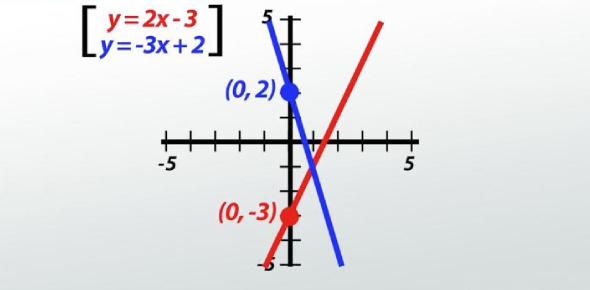Can You Solve These Linear Equations? Trivia Quiz

15 Questions | Total Attempts: 6561Settings• 1.
X + 7 = 10
• A.

4

• B.

3

• C.

-3

• D.

17

• 2.
3x = -12
• A.

-4

• B.

-15

• C.

4

• D.

9

• 3.
3x + x = 12
• A.

4

• B.

-4

• C.

3

• D.

-3

• 4.
-2x = -16
• A.

-14

• B.

8

• C.

14

• D.

-8

• 5.
5x - 3 = 12
• A.

9/5

• B.

4

• C.

5

• D.

3

• 6.
2x + 2 = -14
• A.

6

• B.

-8

• C.

9

• D.

-9

• 7.
6 = -x + 1
• A.

-7

• B.

-6

• C.

-5

• D.

-4

• 8.
5x + 7 = 3x + 13
• A.

10

• B.

5/2

• C.

5

• D.

3

• 9.
10x - 2x + 7 = 2x + 13
• A.

1

• B.

2

• C.

3/5

• D.

-1

• 10.
2(x + 1) = 18
• A.

9

• B.

17/2

• C.

2/17

• D.

8

• 11.
X - 4 = 10 - xenter only your answer (#) in the blank
• 12.Back to top